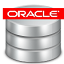What Are the Differences between NUMBER and BINARY_FLOAT

Q

What Are the Differences between NUMBER and BINARY_FLOAT? - Oracle DBA FAQ - Understanding SQL Basics

✍: FYIcenter.com

AThe main differences between NUMBER and BINARY_FLOAT are:

• NUMBER stores values as fixed-point numbers using 1 to 22 bytes.
• BINARY_FLOAT stores values as single precision floating-point numbers.

2007-04-24, 4939👍, 0💬